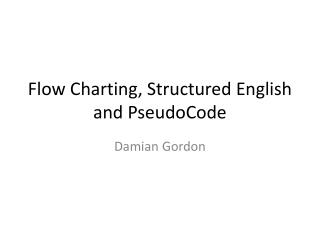DownloadDownload PresentationFlow Charting, Structured English and PseudoCode

# Flow Charting, Structured English and PseudoCode

Download Presentation## Flow Charting, Structured English and PseudoCode

- - - - - - - - - - - - - - - - - - - - - - - - - - - E N D - - - - - - - - - - - - - - - - - - - - - - - - - - -
##### Presentation Transcript

1. Flow Charting, Structured English and PseudoCode Damian Gordon

2. We will recall...

3. Flowcharts • So let’s say we want to express the following algorithm: • Read in a number and print it out.

4. START

5. START Read in A

6. START Read in A Print A

7. START Read in A Print A END

8. Structured English and Pseudocode Structured English Pseudocode PROGRAM PrintNumber: Read A; Print A; END. PROGRAM PrintNumber: Read in a number and print it out. END.

9. Flowcharts • So let’s say we want to express the following algorithm: • Read in a number and print it out double the number.

10. START

11. START Read in A

12. START Read in A Print A*2

13. START Read in A Print A*2 END

14. Structured English and Pseudocode Structured English Pseudocode PROGRAM DoubleNumber: Read A; Print A*2; END. PROGRAM DoubleNumber: Read in a number and print double the number out. END.

15. Or alternatively...

16. START

17. START Read in A

18. START Read in A B = A*2

19. START B = A * 2 can be read as “B gets the value of A multiplied by 2” Read in A B = A*2

20. START Read in A B = A*2 Print B

21. START Read in A B = A*2 Print B END

22. Structured English and Pseudocode Structured English Pseudocode PROGRAM DoubleNumber: Read A; B = A*2; Print B; END. PROGRAM DoubleNumber: Read in a number and print double the number out. END.

23. Flowcharts • So let’s say we want to express the following algorithm: • Read in a number, check if it is odd or even.

24. START

25. START Read in A

26. START Read in A Does A/2 give a remainder?

27. START Read in A Does A/2 give a remainder? Print “It’s Odd” Yes

28. START Read in A Does A/2 give a remainder? Print “It’s Odd” Print “It’s Even” No Yes

29. START Read in A Does A/2 give a remainder? Print “It’s Odd” Print “It’s Even” No Yes END

30. Structured English and Pseudocode Structured English Pseudocode PROGRAM OddOrEven: Read A; IF A/2 gives a remainder THEN Print “It’s Odd”; ELSE Print “It’s Even”; ENDIF; END. PROGRAM OddOrEven: Read in a number. Divide it by two. If there is a remainder, the number is odd, otherwise it’s even. END.

31. Flowcharts • So let’s say we want to express the following algorithm to print out the bigger of two numbers: • Read in two numbers, call them A and B. Is A is bigger than B, print out A, otherwise print out B.

32. START

33. START Read in A and B

34. START Read in A and B A>B?

35. START Read in A and B A>B? Print A Yes

36. START Read in A and B A>B? Print A Print B No Yes

37. START Read in A and B A>B? Print A Print B No Yes END

38. Structured English and Pseudocode Structured English Pseudocode PROGRAM BiggerOfTwo : Read A; IF (A>B) THEN Print A; ELSE Print B; ENDIF; END. PROGRAM BiggerOfTwo: Read in a number. Read in a second number. If the first number is bigger, then print out that number, otherwise print out the other number. END.

39. Flowcharts • So let’s say we want to express the following algorithm to print out the bigger of three numbers: • Read in three numbers, call them A, B and C. • If A is bigger than B, then if A is bigger than C, print out A, otherwise print out C. • If B is bigger than A, then if B is bigger than C, print out B, otherwise print out C.

40. START

41. START Read in A, B and C

42. START Read in A, B and C A>B?

43. START Read in A, B and C A>C? A>B? Yes

44. START Read in A, B and C A>C? A>B? B>C? No Yes

45. START Read in A, B and C A>C? A>B? B>C? No Yes No No Print C

46. START Read in A, B and C A>C? A>B? B>C? No Yes Yes No No Print A Print C

47. START Read in A, B and C A>C? A>B? B>C? No Yes Yes Yes No No Print A Print C Print B

48. START Read in A, B and C A>C? A>B? B>C? No Yes Yes Yes No No Print A Print C Print B END

49. Structured English and Pseudocode Structured English Pseudocode PROGRAM BiggerOfThree: Read A; Read B; Read C; IF A>B THEN IF A>C THEN Print A ELSE Print C END IF; ELSE IF B>C THEN Print B ELSE Print C END IF; END IF; END. PROGRAM BiggerOfThree: Read in a number. Read in a second number. Read in a third number. If the first number is bigger than the second, then if the first number is bigger than the third, then print out the first number, otherwise print out the third number number. If the first number is smaller than the second, then if the second number is bigger than the third, then print out the second number, otherwise print out the third number. END.

50. Flowcharts • So let’s say we want to express the following algorithm: • Print out the numbers from 1 to 5